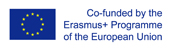This project has been funded with support from the European Commission.
This web site reflects the views only of the author, and the Commission cannot be held responsible for any use which may be made of the information contained therein.

Select language   >   IT EN RO PL LT

### Video Lesson

Subject
Math

Description of the video lesson
In the 2 videos on Pythagorean Theorem students will learn how to:
- Define the Pythagorean theorem
- Solve equations using the Pythagorean Theorem
- Apply the Pythagorean theorem to determine unknown side lengths in right triangles in real-world and mathematical problems in two and three dimensions.

Level of English
Basic English vocabulary

Pre-requisites
Students should have basic English and should also have some knowledge about triangles and angles.

Language aims
Basic English vocabulary linking with mathematical and technical terms.

Key words: Pythagorean Theorem, Pythagoras Theorem
Hypotenuse, triangle, angle, right-angle triangle, square root, leg, side, equation, formula

Subject aims
Upon completion of this lesson, students should be able to:
- Define the Pythagorean theorem
- Solve equations using the Pythagorean Theorem
- Know key vocabulary in English

Students' Difficulties
Students have problems with solving real-life problems and writing the correct equation using the Pythagorean theorem.

Students' Skills
Apply the Pythagorean theorem to determine unknown side lengths in right triangles in real-world and mathematical problems in two and three dimensions.

Two and three dimension images used in conjunction with animation to assist in student understanding of Pythagorean theorem

### Language Skills Developed

- e-book

- Web Site/Portal

- Speaking
- Writing

- Videos

- Listening
- Speaking
- Writing

- Videos

- Listening
- Speaking
- Writing

- Lesson Plans

- Interaction
- Listening
- Speaking
- Writing

- Videos

- Interaction
- Listening
- Speaking

- Exercises

- Speaking
- Writing

- Exercises
- Slides

- Speaking
- Writing

- Exercises

- Listening
- Speaking
- Writing

- Glossary